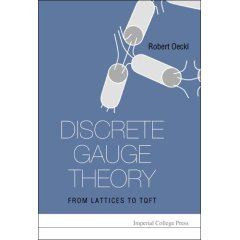## Homepage of Robert Oeckl

 about research book teaching seminars groupI have written a book entitled Discrete Gauge Theory: From Lattices to TQFT. Its main subject is topological quantum field theory and its relation to lattice gauge theory and other models of physics. I extensively use circuit diagrams and a point of view inspired by lattice gauge theory to make the constructions as intuitive and geometrical as possible, especially with a mathematical physicist as a reader in mind. At the same time I sacrifice no mathematical rigour, hopefully making the book attractive also to the interested mathematician. The topological quantum field theories constructed are generalizations of the Turaev-Viro model (in dimension three) and the Crane-Yetter model (in dimension four). The construction is first performed as a generalization of lattice gauge theory using quantum groups and then specialized to the topological case. This is done using cellular moves, a generalization of Pachner moves to cellular decompositions of manifolds. Furthermore, the construction is performed first in a way applicable to manifolds of arbitrary dimension. Then, the low dimensional situation (2,3,4) is adapted to the specialities of topology in that dimension. This goes along with a corresponding choice of category. Applications discussed in the book include spin foam models of quantum gravity and quantum supergravity, non-abelian duality, spin models, generating field theory (generalized matrix models) and renormalization.

Last updated 1 October 2008.